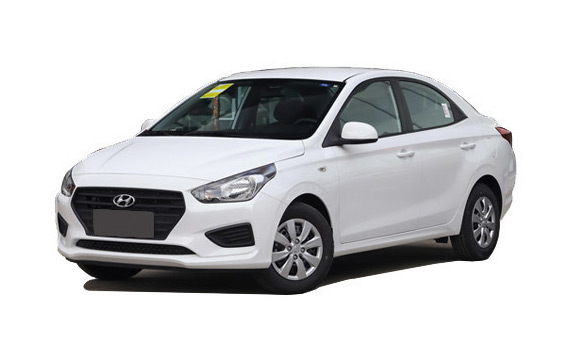# 瑞纳本地参考价： 4.99 万元起 厂商指导价：4.99-7.39 万
 车市行情　｜　瑞纳 20201.4L 手动焕彩版降0.00万
 最新年款： 2020款 级　别：小型车 结　构：4门5座三厢车 车身颜色： 红色 油　耗：6.8L/100km 排　量：1.4 内饰配色： 红黑 保　修：3年或6万公里 变速箱：5挡手动|4挡自动

#### 悦纳&瑞纳“双A”CP，颜艺双绝获百万粉丝70kW(1.4L自然吸气) 自然吸气 官方指导价 本地参考价 车型信息 2020款 1.4L 手动焕新版 手动变速箱 前置前驱 4.99万暂无 参数　图片　＋对比 2020款 1.4L 手动焕彩版 手动变速箱 前置前驱 5.49万暂无 参数　图片　＋对比 2020款 1.4L 手动焕彩天窗版 手动变速箱 前置前驱 5.79万暂无 参数　图片　＋对比 2020款 1.4L 手动焕彩网联版 手动变速箱 前置前驱 5.79万暂无 参数　图片　＋对比 2020款 1.4L 自动跃享版 4挡自动变速箱 前置前驱 6.59万暂无 参数　图片　＋对比 2020款 1.4L 自动跃享网联版 4挡自动变速箱 前置前驱 6.89万暂无 参数　图片　＋对比 2020款 1.4L 自动跃劲版 4挡自动变速箱 前置前驱 7.39万暂无 参数　图片　＋对比

### 绕车实拍 外观 中控 座椅 细节 全部### 瑞纳 新闻资讯#### 新悦纳&全新瑞纳秒杀同级#### 悦纳&瑞纳“双A”CP，颜艺双绝获百万粉丝#### 全新瑞纳获奖 直抵锋范

10万块钱在买车人手中,是夜以继日辛勤付出才挣到的辛苦钱,每一分都值得被尊重。买一辆中高配的合资车更踏实!从颜值、经济性、用车成本、品牌溢...#### 瑞纳VS锋范 谁才是“人生第一款车”最优解#### 全新一代悦纳购车手册看过来#### 买车不纠结 锋范VS瑞纳的深度评测来了#### 带你解锁全新瑞纳“会说话”的黑科技#### 全新瑞纳抢占互联网汽车入口

9月19日，北京现代宣布全新瑞纳正式上市，4.99-7.39万元的售价或将横扫入门级家轿市场，因此这也将是北京现代今年下半年最值得关注的大手笔！...#### 有台会说话的全新瑞纳 再不怕开车没激情#### 嘴甜会“撩”的智能小车 扒一扒全新瑞纳的智能黑科技#### 随时随地畅聊全新瑞纳这套智能网联系统不一般#### 会说话的全新瑞纳到店 崇尚潮流的你还不来吗#### 五万元唯一可选的合资精品 全新瑞纳即将上市#### 全新瑞纳要搞事情 智能语音系统竟比iPhone8更让人期待#### 现代这套智能网联帅炸 全新瑞纳方言操控无障碍#### 好看好用还不贵 全新瑞纳重新定义入门级家轿

8月25日成都车展，北京现代正式公布了全新瑞纳预售价为5~8万元，并将于9月上市。消息一出，北京现代的展台更是人满为患。究竟是什么原因让全新...#### 升级！试驾体验北京现代VERNA瑞纳#### C-NCAP碰撞 现代瑞纳以47分获得五星

C-NCAP碰撞现代瑞纳以47分获得五星。#### 实拍2014款北京现代瑞纳 整体变化不大#### 瑞纳现金优惠3900元 部分车型现车销售#### 北京现代新款瑞纳上市 售7.39-10.69万#### 新款瑞纳三厢价格曝光 售7.39-10.69万### 猜你喜欢

﻿
• 快速找车
• 选择品牌
• 选择品牌
• A  奥迪
• A  阿斯顿·马丁
• A  阿尔法·罗密欧
• B  宝沃
• B  布加迪
• B  巴博斯
• B  保时捷
• B  宾利
• B  奔驰
• B  宝马
• B  本田
• B  别克
• B  标致
• B  比亚迪
• B  宝骏
• B  北汽制造
• B  北汽新能源
• B  北汽幻速
• B  北汽威旺
• B  北京汽车
• B  奔腾
• B  北汽绅宝
• B  北汽昌河
• C  长安欧尚
• C  长安
• C  长安凯程
• C  长城
• D  大众
• D  道奇
• D  DS
• D  东南
• D  东风风神
• D  东风风行
• D  东风小康
• D  东风风度
• D  东风
• F  福特
• F  丰田
• F  菲亚特
• F  法拉利
• F  福田
• F  福迪
• F  福汽启腾
• G  观致
• G  广汽传祺
• G  广汽吉奥
• G  GMC
• H  红旗
• H  汉腾汽车
• H  哈弗
• H  哈飞
• H  海格
• H  海马
• H  华颂
• H  黄海
• H  华泰
• H  恒天
• J  捷途
• J  几何汽车
• J  捷达
• J  吉利汽车
• J  捷豹
• J  Jeep
• J  江淮
• J  江铃
• J  金杯
• J  九龙
• J  金旅
• K  凯翼
• K  凯迪拉克
• K  克莱斯勒
• K  科尼塞克
• K  卡威
• K  开瑞
• L  路虎
• L  林肯
• L  劳斯莱斯
• L  兰博基尼
• L  雷克萨斯
• L  铃木
• L  领克
• L  雷诺
• L  理念
• L  力帆
• L  莲花汽车
• L  猎豹
• L  路特斯
• L  陆风
• M  马自达
• M  MG
• M  MINI
• M  玛莎拉蒂
• M  摩根
• M  迈凯轮
• N  纳智捷
• O  欧拉
• O  欧宝
• O  讴歌
• O  欧朗
• Q  奇瑞
• Q  起亚
• Q  启辰
• R  日产
• R  荣威
• R  瑞麒
• S  SERES赛力斯
• S  三菱
• S  斯威汽车
• S  萨博
• S  smart
• S  斯柯达
• S  斯巴鲁
• S  思铭
• S  双龙
• S  上汽大通
• S  双环
• T  特斯拉
• T  腾势
• W  蔚来
• W  沃尔沃
• W  WEY
• W  五菱汽车
• W  五十铃
• W  威兹曼
• W  威麟
• X  现代
• X  雪佛兰
• X  星途
• X  雪铁龙
• X  小鹏汽车
• X  西雅特
• Y  一汽
• Y  英菲尼迪
• Y  英致
• Y  依维柯
• Y  野马汽车
• Y  永源
• Z  众泰
• Z  中华
• Z  中兴
• Z  知豆
• 选择车系
• 选择车系
• 车型对比
• 选择品牌
• 选择品牌
• A  奥迪
• A  阿斯顿·马丁
• A  阿尔法·罗密欧
• B  宝沃
• B  布加迪
• B  巴博斯
• B  保时捷
• B  宾利
• B  奔驰
• B  宝马
• B  本田
• B  别克
• B  标致
• B  比亚迪
• B  宝骏
• B  北汽制造
• B  北汽新能源
• B  北汽幻速
• B  北汽威旺
• B  北京汽车
• B  奔腾
• B  北汽绅宝
• B  北汽昌河
• C  长安欧尚
• C  长安
• C  长安凯程
• C  长城
• D  大众
• D  道奇
• D  DS
• D  东南
• D  东风风神
• D  东风风行
• D  东风小康
• D  东风风度
• D  东风
• F  福特
• F  丰田
• F  菲亚特
• F  法拉利
• F  福田
• F  福迪
• F  福汽启腾
• G  观致
• G  广汽传祺
• G  广汽吉奥
• G  GMC
• H  红旗
• H  汉腾汽车
• H  哈弗
• H  哈飞
• H  海格
• H  海马
• H  华颂
• H  黄海
• H  华泰
• H  恒天
• J  捷途
• J  几何汽车
• J  捷达
• J  吉利汽车
• J  捷豹
• J  Jeep
• J  江淮
• J  江铃
• J  金杯
• J  九龙
• J  金旅
• K  凯翼
• K  凯迪拉克
• K  克莱斯勒
• K  科尼塞克
• K  卡威
• K  开瑞
• L  路虎
• L  林肯
• L  劳斯莱斯
• L  兰博基尼
• L  雷克萨斯
• L  铃木
• L  领克
• L  雷诺
• L  理念
• L  力帆
• L  莲花汽车
• L  猎豹
• L  路特斯
• L  陆风
• M  马自达
• M  MG
• M  MINI
• M  玛莎拉蒂
• M  摩根
• M  迈凯轮
• N  纳智捷
• O  欧拉
• O  欧宝
• O  讴歌
• O  欧朗
• Q  奇瑞
• Q  起亚
• Q  启辰
• R  日产
• R  荣威
• R  瑞麒
• S  SERES赛力斯
• S  三菱
• S  斯威汽车
• S  萨博
• S  smart
• S  斯柯达
• S  斯巴鲁
• S  思铭
• S  双龙
• S  上汽大通
• S  双环
• T  特斯拉
• T  腾势
• W  蔚来
• W  沃尔沃
• W  WEY
• W  五菱汽车
• W  五十铃
• W  威兹曼
• W  威麟
• X  现代
• X  雪佛兰
• X  星途
• X  雪铁龙
• X  小鹏汽车
• X  西雅特
• Y  一汽
• Y  英菲尼迪
• Y  英致
• Y  依维柯
• Y  野马汽车
• Y  永源
• Z  众泰
• Z  中华
• Z  中兴
• Z  知豆
• 选择车系
• 选择车系
• 选择车型
• 选择车型
• 意见反馈